# Bihar Board 12th Maths Objective Answers Chapter 10 Vector Algebra

Bihar Board 12th Maths Objective Questions and Answers

## Bihar Board 12th Maths Objective Answers Chapter 10 Vector Algebra

Question 1.
If $$\left(\frac{1}{2}, \frac{1}{3}, n\right)$$ are the direction cosines of a line, then the value of n is
(a) $$\frac{\sqrt{23}}{6}$$
(b) $$\frac{23}{6}$$
(c) $$\frac{2}{3}$$
(d) $$\frac{3}{2}$$
(a) $$\frac{\sqrt{23}}{6}$$

Question 2.
Find the magnitude of vector $$3 \hat{i}+2 \hat{j}+12 \hat{k}$$.
(a) √157
(b) 4√11
(c) √213
(d) 9√3
(a) √157

Direction (3 – 5): Study the given parallelogram and answer the following questions.Question 3.
Which of the following represents equal vectors?
(a) a, c
(b) b, d
(c) b, c
(d) m, d
(b) b, d

Question 4.
Which of the following represents collinear but not equal vectors?
(a) a, c
(b) b, d
(c) b, m
(d) Both (a) and (b)
(a) a, c

Question 5.
Which of the following represents coinitial vector?
(a) c, d
(b) m, b
(c) b, d
(d) Both (a) and (b)
(d) Both (a) and (b)

Question 6.
The unit vector in the direction of the sum of vectors(a) $$\frac{1}{5 \sqrt{2}}(3 \hat{i}+4 \hat{j}+5 \hat{k})$$

Question 7.
The vectors $$3 \hat{i}+5 \hat{j}+2 \hat{k}, 2 \hat{i}-3 \hat{j}-5 \hat{k}$$ and $$5 \hat{i}+2 \hat{j}-3 \hat{k}$$ form the sides of
(a) Isosceles triangle
(b) Right triangle
(c) Scalene triangle
(d) Equilaterala triangle
(d) Equilaterala triangle

Question 8.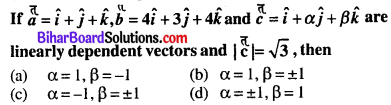(d) α = ±1, β = 1

Question 9.
The vectors $$a=x \hat{i}-2 \hat{j}+5 \hat{k}$$ and $$b=\hat{i}+y \hat{j}-z \hat{k}$$ are collinear, if
(a) x =1, y = -2, z = -5
(b) x= 1.2, y = -4, z = -10
(c) x = -1/2, y = 4, z = 10
(d) All of these
(d) All of these

Question 10.
The vector $$\hat{i}+x \hat{j}+3 \hat{k}$$ is rotated through an angle θ and doubled in magnitude, then it becomes $$4 \hat{i}+(4 x-2) \hat{i}+2 \hat{k}$$. The value of x is
(a) $$\left\{-\frac{2}{3}, 2\right\}$$
(b) $$\left\{\frac{1}{3}, 2\right\}$$
(c) $$\left\{\frac{2}{3}, 0\right\}$$
(d) {2, 7}
(a) $$\left\{-\frac{2}{3}, 2\right\}$$

Question 11.
Three points (2, -1, 3), (3, -5, 1)and (-1, 11, 9) are
(a) Non-collinear
(b) Non-coplanar
(c) Collinear
(d) None of these
(c) CollinearQuestion 12.
The points with position vectors $$60 \hat{i}+3 \hat{j}, 40 \hat{i}-8 \hat{j}$$ and $$a \hat{i}-5 \hat{j}$$ are collinear if
(a) a = -40
(b) a = 40
(c) a = 20
(d) None of these
(a) a = -40

Question 13.
The position vectors of the points A, B, C are $$(2 \hat{i}+\hat{j}-\hat{k}),(3 \hat{i}-2 \hat{j}+\hat{k})$$ and $$(\hat{i}+4 \hat{j}-3 \hat{k})$$ respectively. These points
(a) form an isosceles triangle
(b) form a right angled triangle
(c) are collinear
(d) form a scalene triangle
(a) form an isosceles triangle

Question 14.
The figure formed by the four points $$\hat{i}+\hat{j}-\hat{k}$$, $$2 \hat{i}+3 \hat{j}, 5 \hat{j}-2 \hat{k}$$ and $$\hat{k}-\hat{j}$$ is
(a) trapezium
(b) rectangle
(c) parallelogram
(d) None of these
(d) None of these

Question 15.
If x coordinate of a point P of a line joining the points Q(2, 2, 1) and R(5, 2, -2) is 4, then the z coordinate of P is
(a) -2
(b) -1
(c) 1
(d) 2
(b) -1Question 16.
If O is origin and C is the mid point of A(2, -1) and B(-4, 3), then the value of OC is
(a) $$\hat{i}+\hat{j}$$
(b) $$\hat{i}-\hat{j}$$
(c) $$-\hat{i}+\hat{j}$$
(d) $$-\hat{i}-\hat{j}$$
(c) $$-\hat{i}+\hat{j}$$

Question 17.
The vectors AB = $$3 \hat{i}+4 \hat{k}$$ and AC = $$A C=5 \hat{i}-2 \hat{j}+4 \hat{k}$$ are the side of a ΔABC. The length of the median through A is
(a) √18
(b) √72
(c) √33
(d) √288
(c) √33

Question 18.
The summation of two unit vectors is a third unit vector, then the modulus of the difference of the unit vector is
(a) √3
(b) 1 – √3
(c) 1 + √3
(d) -√3
(a) √3

Question 19.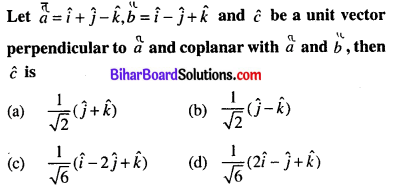(d) $$\frac{1}{\sqrt{6}}(2 \hat{i}-\hat{j}+\hat{k})$$

Question 20.(c) $$\pi \geq \theta>\frac{2 \pi}{3}$$

Question 21.
Let a, b and c be vectors with magnitudes 3, 4 and 5 respectively and a + b + c = 0, then the values of a.b + b.c + c.a is
(a) 47
(b) 25
(c) 50
(d) -25
(d) -25

Question 22.
If |a| = |b| = 1 and |a + b| = √3, then the value of (3a – 4b).(2a + 5b) is
(a) -21
(b) $$-\frac{21}{2}$$
(c) 21
(d) $$\frac{21}{2}$$
(b) $$-\frac{21}{2}$$

Question 23.(c) $$\frac{1}{\sqrt{2}}(\hat{i}+\hat{j})$$

Question 24.
If |a – b| = |a| = |b| = 1, then the angle between a and b is
(a) $$\frac{\pi}{3}$$
(b) $$\frac{3 \pi}{4}$$
(c) $$\frac{\pi}{2}$$
(d) 0
(a) $$\frac{\pi}{3}$$

Question 25.(d) |a|2

Question 26.
a, b, c are three vectors, such that a + b + c = 0, |a|= 1, |b|= 2, |c|= 3, then a.b + b.c + c is equal to
(a) 0
(b) -7
(c) 7
(d) 1
(b) -7

Question 27.
If |a + b| = |a – b|, then angle between a and b is (a ≠ 0, b ≠ 0)
(a) $$\frac{\pi}{3}$$
(b) $$\frac{\pi}{6}$$
(c) $$\frac{\pi}{4}$$
(d) $$\frac{\pi}{2}$$
(d) $$\frac{\pi}{2}$$

Question 28.
If a and b are two unit vectors inclined to x-axis at angles 30° and 120° respectively, then |a + b| equals
(a) $$\sqrt{\frac{2}{3}}$$
(b) √2
(c) √3
(d) 2
(d) 2Question 29.
If the angle between $$\hat{i}+\hat{k}$$ and $$\hat{i}+\hat{j}+a \hat{k}$$ is $$\frac{\pi}{3}$$, then the value of a is
(a) 0 or 2
(b) -4 or 0
(c) 0 or -3
(d) 2 or -2
(b) -4 or 0

Question 30.
The length of longer diagronai of the parallelogram constructed on 5a + 2b and a – 3b. If it is given that
|a| = 2√2, |b| = 3 and angle between a and b is $$\frac{\pi}{4}$$, is
(a) 15
(b) √113
(c) √593
(d) √369
(c) √593

Question 31.
If a, b, c are unit vectors, then |a – b| + |b – c| + |c – a| does not exceed
(a) 4
(b) 9
(c) 8
(d) 6
(b) 9

Question 32.
Find the value of λ so that the vectors $$2 \hat{i}-4 \hat{j}+\hat{k}$$ and $$4 \hat{i}-8 \hat{j}+\lambda \hat{k}$$ are perpendicular.
(a) -15
(b) 10
(c) -40
(d) 20
(c) -40

Question 33.
The dot product of a vector with the vectors $$\hat{i}+\hat{j}-3 \hat{k}, \hat{i}+3 \hat{j}-2 \hat{k}$$ and $$2 \hat{i}+\hat{j}+4 \hat{k}$$ are 0, 5 and 8 respectively. Find the vector.
(a) $$\hat{i}+2 \hat{j}+\hat{k}$$
(b) $$-\hat{i}+3 \hat{j}-2 \hat{k}$$
(c) $$\hat{i}+2 \hat{j}+3 \hat{k}$$
(d) $$\hat{i}-3 \hat{j}-3 \hat{k}$$
(a) $$\hat{i}+2 \hat{j}+\hat{k}$$Question 34.
If a, b, c are three mutually perpendicular vectors of equal magnitude, find the angle between a and a + b + c.
(a) $$\cos ^{-1}(1 / \sqrt{3})$$
(b) $$\cos ^{-1}(1 / 2 \sqrt{2})$$
(c) $$\cos ^{-1}(1 / 3 \sqrt{3})$$
(d) $$\cos ^{-1}(1 / 2 \sqrt{3})$$
(a) $$\cos ^{-1}(1 / \sqrt{3})$$

Question 35.
Find the angle between the vectors a + b and a – b if a = $$2 \hat{i}-\hat{j}+3 \hat{k}$$ and b = $$b=3 \hat{i}+\hat{j}-2 \hat{k}$$
(a) $$\frac { \pi }{ 6 }$$
(b) $$\frac { \pi }{ 3 }$$
(c) $$\frac { \pi }{ 2 }$$
(d) 0
(c) $$\frac { \pi }{ 2 }$$

Question 36.
If a = $$2 \hat{i}+\hat{j}+2 \hat{k}$$ and b = $$5 \hat{i}-3 \hat{j}+\hat{k}$$, then the projection of b on a is
(a) 3
(b) 4
(c) 5
(d) 6
(a) 3

Question 37.
Let $$a=\hat{i}+2 \hat{j}+\hat{k}, b=\hat{i}-\hat{j}+\hat{k}, c=\hat{i}+\hat{j}-\hat{k}$$. A vector coplanar to a and b has a projection along c of magnitude $$\frac{1}{\sqrt{3}}$$, then the vector is
(a) $$4 \hat{i}-\hat{j}+4 \hat{k}$$
(b) $$4 \hat{i}+\hat{j}-4 \hat{k}$$
(c) $$2 \hat{i}+\hat{j}+\hat{k}$$
(d) None of these
(a) $$4 \hat{i}-\hat{j}+4 \hat{k}$$

Question 38.
The component of i in the direction of the vector $$\hat{i}+\hat{j}+2 \hat{k}$$ is
(a) √6
(b) 6
(c) 6√6
(d) $$\frac{\sqrt{6}}{6}$$
(d) $$\frac{\sqrt{6}}{6}$$Question 39.
Find the projection of b + c on a where a = $$\hat{i}+2 \hat{j}+\hat{k}$$, b = $$\hat{i}+3 \hat{j}+\hat{k}$$ and c = $$\hat{i}+\hat{k}$$.
(a) $$\frac { 5 }{ \surd 3 }$$
(b) 2√2
(c) $$\frac { 3 }{ \surd 2 }$$
(d) $$\frac { 10 }{ \surd 6 }$$
(d) $$\frac { 10 }{ \surd 6 }$$

Question 40.
If a = $$\hat{i}+\hat{j}+\hat{k}$$, b = $$\hat{i}+3 \hat{j}+5 \hat{k}$$ and c = $$7 \hat{i}+9 \hat{j}+11 \hat{k}$$, then the area of parallelogram having diagonals a + b and b + c is
(a) 4√6
(b) $$\frac{1}{2} \sqrt{21}$$
(c) $$\frac{\sqrt{6}}{2}$$
(d) √6
(a) 4√6

Question 41.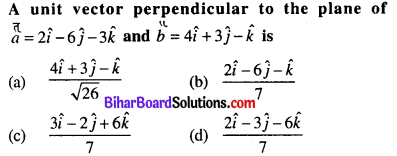(c) $$\frac{3 \hat{i}-2 \hat{j}+6 \hat{k}}{7}$$

Question 42.
The area of parallelogram whose adjacent sides are $$\hat{i}-2 \hat{j}+3 \hat{k}$$ and $$2 \hat{i}+\hat{j}-4 \hat{k}$$ is
(a) 10√6
(b) 5√6
(c) 10√3
(d) 5√3
(b) 5√6

Question 43.
If AB × AC = $$2 \hat{i}-4 \hat{j}+4 \hat{k}$$, then the are of ΔABC is
(a) 3 sq. units
(b) 4 sq. units
(c) 16 sq. units
(d) 9 sq. units
(a) 3 sq. units

Question 44.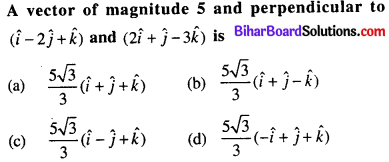(a) $$\frac{5 \sqrt{3}}{3}(\hat{i}+\hat{j}+\hat{k})$$

Question 45.
|a × b|2 + |a.b|2 = 144 and |a| = 4, then |b| is equal to
(a) 12
(b) 3
(c) 8
(d) 4
(b) 3

Question 46.
If |a × b| = 4 and |a.b| = 2, then |a|2 |b|2 is equal to
(a) 2
(b) 6
(c) 8
(d) 20
(d) 20

Question 47.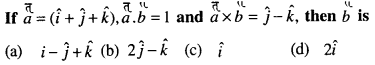(c) $$\hat{i}$$

Question 48.
The two vectors a = $$2 \hat{i}+\hat{j}+3 \hat{k}$$ and b = 4 \hat{i}-\lambda \hat{j}+6 \hat{k} ae parallel, if λ is equal to
(a) 2
(b) -3
(c) 3
(d) 2
(d) 2

Question 49.
If |a|= 5, |b|= 13 and |a × b|= 25, find a.b
(a) ±10
(b) ±40
(c) ±60
(d) ±25
(c) ±60

Question 50.
Find the value of λ so that the vectors $$2 i-4 \hat{j}+\hat{k}$$ and $$4 i-8 \hat{j}+\lambda \hat{k}$$ are parallel.
(a) -1
(b) 3
(c) -4
(d) 2
(d) 2Question 51.
If a + b + c = 0, then a × b =
(a) c × a
(b) b × c
(c) 0
(d) Both (a) and (b)
(d) Both (a) and (b)

Question 52.
If a is perpendicular to b and c, |a| = 2, |b| = 3, |c| = 4 and the angle between b and c is $$\frac{2 \pi}{3}$$, |abc| is equal to
(a) 4√3
(b) 6√3
(c) 12√3
(d) 18√3
(c) 12√3

Question 53.(b) a

Question 54.(a) neither x nor y

Question 55.
If a, b, c are three non-coplanar vectors, then (a + b + c).[(a + b) × (a + c)] is
(a) 0
(b) 2[abc]
(c) -[abc]
(d) [abc]
(c) -[abc]

Question 56.
If u, v and w are three non-coplanar vectors, then (u + v – w).[(u – v) × (v – w)] equals
(a) 0
(b) u.v × w
(c) u.w × v
(d) 3u.v × w
(b) u.v × w

Question 57.
If unit vector c makes an angle $$\frac{\pi}{3}$$ with $$\hat{i} \times \hat{j}$$, then minimum and maximum values of $$(\hat{i} \times \hat{j}) \cdot c$$ respectively are
(a) 0, $$\frac{\sqrt{3}}{2}$$
(b) $$-\frac{\sqrt{3}}{2}, \frac{\sqrt{3}}{2}$$
(c) -1, $$\frac{\sqrt{3}}{2}$$
(d) None of these
(b) $$-\frac{\sqrt{3}}{2}, \frac{\sqrt{3}}{2}$$Question 58.
The volume of the tetrahedron whose conterminous edges are $$\hat{j}+\hat{k}, \hat{i}+\hat{k}, i+\hat{j}$$ is
(a) $$\frac{1}{6}$$ cu. unit
(b) $$\frac{1}{3}$$ cu. unit
(c) $$\frac{1}{2}$$ cu. unit
(d) $$\frac{2}{3}$$ cu. unit
(b) $$\frac{1}{3}$$ cu. unit

Question 59.
If the vectors $$2 \hat{i}-3 \hat{j}, i+\hat{j}-\hat{k}$$ and $$3 \hat{i}-\hat{k}$$ form three concurrent edges of a parallelopiped, then the volume of the parallelopiped is
(a) 8
(b) 10
(c) 4
(d) 14
(c) 4

Question 60.
The volume of the parallelopiped whose edges are represented by $$-12 \hat{i}+\alpha \hat{k}, 3 j-\hat{k}$$ and $$2 \hat{i}+j-15 \hat{k}$$ is 546 cu. units. Then α =
(a) 3
(b) 2
(c) -3
(d) -2
(c) -3

Question 61.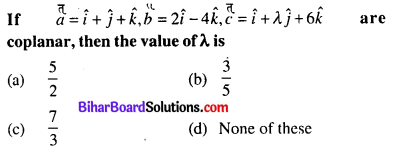(d) None of these

Question 62.(a) -2

Question 63.(a) all values of x

Question 64.
If the vectors $$\hat{i}-2 \hat{j}+3 \hat{k},-2 \hat{i}+3 \hat{j}-4 \hat{k}, \lambda \hat{i}-\hat{j}+2 \hat{k}$$ are coplanar, then the value of λ is equal to
(a) 0
(b) 1
(c) 2
(d) 3
(a) 0

Question 65.
Find the value of λ if the vectors, a = $$2 \hat{i}-\hat{j}+\hat{k}$$, b = $$\hat{i}+2 \hat{j}-3 \hat{k}$$ and c = $$3 \hat{i}-\lambda \hat{j}+5 \hat{k}$$ are coplanar.
(a) 4
(b) -2
(c) -6
(d) 5
(a) 4Question 66.
Find λ if the vectors $$\hat{i}-\hat{j}+\hat{k}, 3 \hat{i}+\hat{j}+2 \hat{k}$$ and $$\hat{i}+\lambda \hat{j}-\hat{k}$$ are coplanar.
(a) 5
(b) 12
(c) 15
(d) 8
(c) 15

Question 67.
The vector in the direction of the vector $$\hat{i}-2 \hat{j}+2 \hat{k}$$ that has magnitude 9 is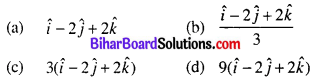(c) $$3(\hat{i}-2 \hat{j}+2 \hat{k})$$

Question 68.
The position vector of the point which divides the join of points 2a – 3b and a + b in the ratio 3 : 1 is(d) $$\frac{5 a}{4}$$

Question 69.
The angle between two vectors a and b with magnitudes √3 and 4, respectively and a.b = 2√3 is
(a) $$\frac{\pi}{6}$$
(b) $$\frac{\pi}{3}$$
(c) $$\frac{\pi}{2}$$
(d) $$\frac{5 \pi}{2}$$
(b) $$\frac{\pi}{3}$$

Question 70.
Find the value of λ such that the vectors a = $$2 \hat{i}+\lambda \hat{j}+\hat{k}$$ and b = $$\hat{i}+2 \hat{j}+3 \hat{k}$$ are orthogonal.
(a) 0
(b) 1
(c) $$\frac{3}{2}$$
(d) $$-\frac{5}{2}$$
(d) $$-\frac{5}{2}$$Question 71.
The value of λ for which the vectors $$3 \hat{i}-6 \hat{j}+\hat{k}$$ and $$2 \hat{i}-4 \hat{j}+\lambda \hat{k}$$ are parallel is
(a) $$\frac{2}{3}$$
(b) $$\frac{3}{2}$$
(c) $$\frac{5}{2}$$
(d) $$\frac{2}{5}$$
(a) $$\frac{2}{3}$$

Question 72.
The vectors from origin to the points A and B are a = $$2 \hat{i}-3 \hat{j}+2 \hat{k}$$ and b = $$2 \hat{i}+3 \hat{j}+\hat{k}$$, respectively then the area of triangle OAB is
(a) 340
(b) √25
(c) √229
(d) $$\frac{1}{2}$$ √229
(d) $$\frac{1}{2}$$ √229

Question 73.
The vectors $$\lambda \hat{i}+\hat{j}+2 \hat{k}, \hat{i}+\lambda \hat{j}-\hat{k}$$ and $$2 \hat{i}-\hat{j}+\lambda \hat{k}$$ are coplanar if
(a) λ = -2
(b) λ = 0
(c) λ = 1
(d) λ = -1
(a) λ = -2

Question 74.
If a, b, c are unit vectors such that a + b + c = 0, then the value of a.b + b.c + c.a is
(a) 1
(b) 3
(c) $$-\frac{3}{2}$$
(d) None of these
(c) $$-\frac{3}{2}$$

Question 75.
If |a| = 4 and -3 ≤ λ ≤ 2, then the range of |λa| is
(a) [0, 8]
(b) [-12, 8]
(c) [0, 12]
(d) [8, 12]The number of vectors of unit length perpendicular to the vectors a = $$2 \hat{i}+\hat{j}+2 \hat{k}$$ and b = $$\hat{j}+\hat{k}$$ is